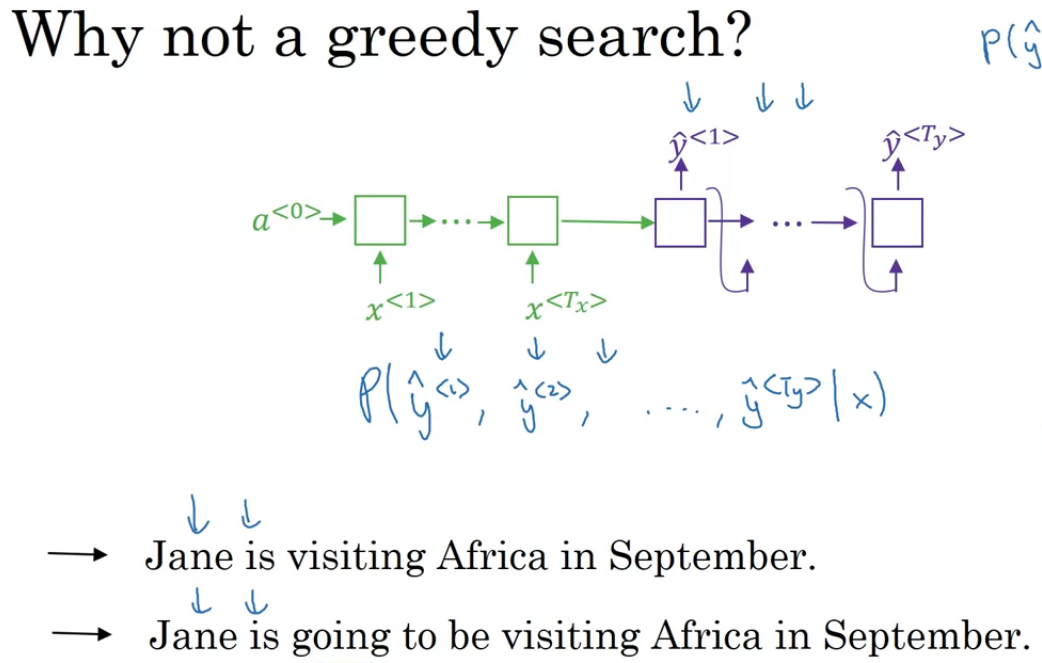Sequence models → Week 03 (Attention mechanism)

In Language Model, we find probability of sentence.

Decoder in Machine Translation system is same as Language Model and a<0> in language model is similar to Encoder in Machine Translation.

In M/C Translation, we use beam search instead of greedy search.P(Jane is going/X) > P(Jane is visiting/X) but sentence 1 is more optimial

Beam Search

Beam Width considered is 3 i.e. Top 3 words will be considered as candidate..

Say, Word1= “in”, need to find P(Y2/X,”in”) i.e. Prob. of Y2 given X and “in”.

Log is strictly monotonically increasing function i.e. maximizing P(Y/X) is same as maximizing Log (p(Y/X)) ..

Above P(Yt/X,Y1,……,Yt-1), Unnaturally tends/prefer short translations as multiplying no less than 1 will give short tiny number ..

Attention (Alpha (t,x))→ How much weight to be used for generating t word using time-stamp x

Part — 01 (Attention) → a is combination of backward and forward propagation .. For 1st word, will have 5 timestamp alphas i.e. attention weights and its summation will be 1. C (Context Vectors) is summation of different timestamps.

PART — 02 (Attention) → A

Now, how to calculate Alpha (t,t’) i.e. Amount of attention Y(t) should pay to a(t’).